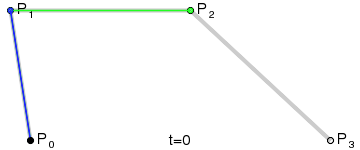# Bezier Curve

## Description

“Bezier Curve” is a method of generating smooth curve in computer graphics. Note that it is a method of curve representation, and not a curve in the usual sense in math contexts.

The Bezier Curve method is named after the engineer Pierre Bézier.

Given 4 points P0, P1, P2, P3, find a parametric formula in polynomial such that it passes P0 with tangent vector[P0,P1], and passes P2 with tangent vector[P3,P2].

Solution, written in complex number notation:

`z[t]=(1-t)^3*P0 + 3*t(1-t)^2*P1 + 3*t^2(1-t)*P2 + t^3*P3`

We verify that

```z==P0, z'==3*(P1-P0)

z=P2, z'==3*(P2-P3)```Bézier curve generation

Cubic Bezier Curve

see Bézier curve

2006-05

If you have a question, put \$5 at patreon and message me.